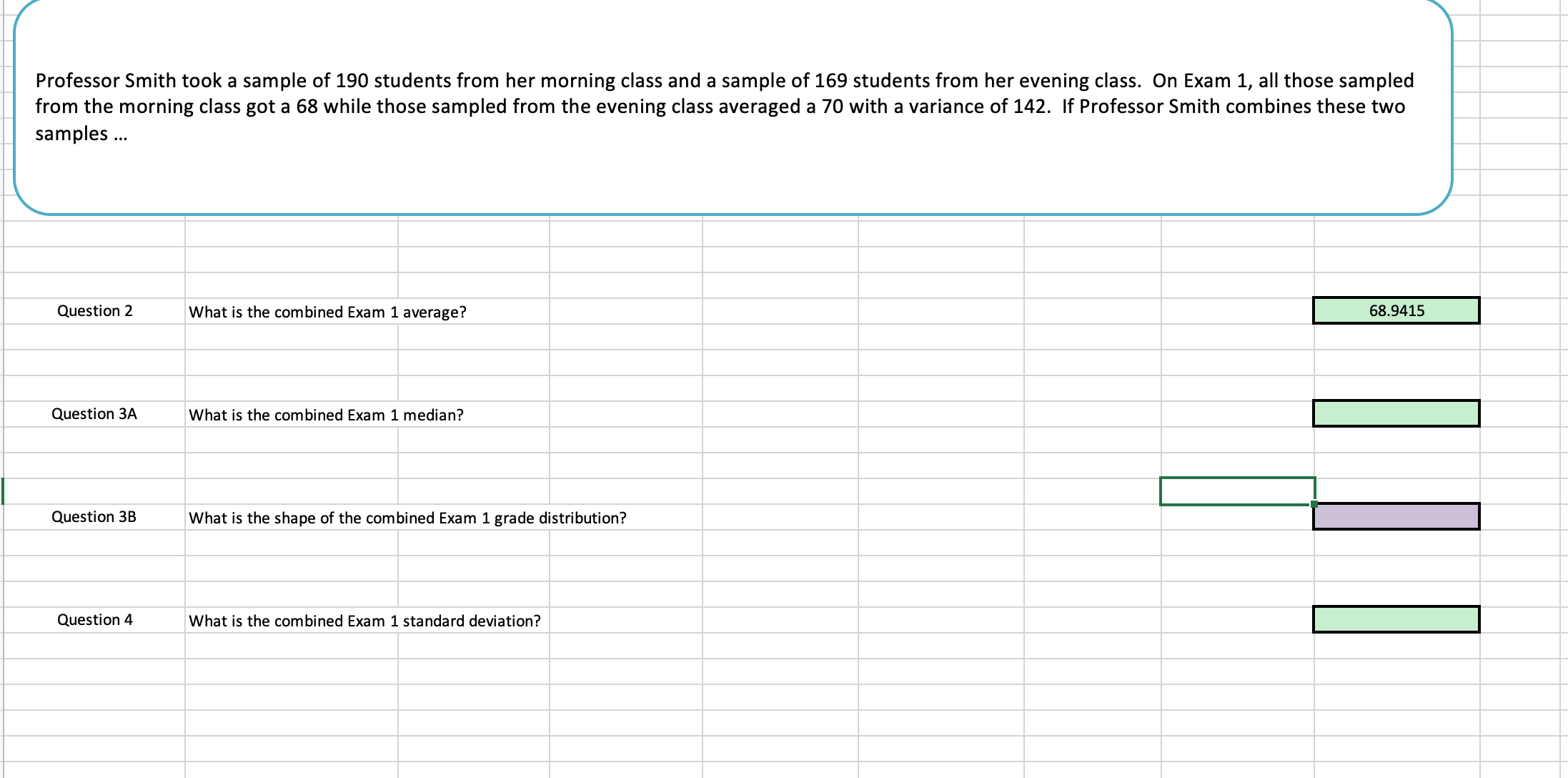# Professor Smith took a sample of 190 students from her morning class and a sample of 169 students from her evening class. On Exam 1, all those sampledfrom the morning class got a 68 while those sampled from the evening class averaged a 70 with a variance of 142. If Professor Smith combines these twosamples..Question 2What is the combined Exam 1 average?68.9415Question 3AWhat is the combined Exam 1 median?Question 3BWha is the shape of the combined Exam 1 grade distribution?Question 4What is the combined Exam 1 standard deviation?

Question
1227 views

How to solve for question 2-4?help_outlineImage TranscriptioncloseProfessor Smith took a sample of 190 students from her morning class and a sample of 169 students from her evening class. On Exam 1, all those sampled from the morning class got a 68 while those sampled from the evening class averaged a 70 with a variance of 142. If Professor Smith combines these two samples.. Question 2 What is the combined Exam 1 average? 68.9415 Question 3A What is the combined Exam 1 median? Question 3BWha is the shape of the combined Exam 1 grade distribution? Question 4 What is the combined Exam 1 standard deviation? fullscreen
check_circle

Step 1

Question 2: Sample statistics of the two samples:

The given statistics for the two randomly selected samples regarding the data of Exam 1 scores of morning class and evening class students for Professor Smith are:

The number of students from the morning class is n1 = 190.

The number of students from the evening class is n2 = 169.

The average score in Exam 1 for the students from evening class is x2bar = 70.

The variance scores of Exam 1 for the students from evening class is s2^2 = 142.

Furthermore, it is given that each student from the morning class has scored 68 in Exam 1.

Mean: Mean is an important measure of center when the data is quantitative. Mean of a data set is the sum of the data set divided by the size.

The average score in Exam 1 for the students from morning class:

Step 2

Obtain the combined Exam 1 score average.

The average score in Exam 1 for the students from morning class is x1bar = 68 and the average score in Exam 1 for the students from evening class is x2bar = 70.

The combined Exam 1 score average is obtained as xbar = 68.942 from the calculation given below:

Step 3

Question 3A: Obtain the combined Exam 1 score median.

Median: The medianis the middle value in an ordered sequence of data. If there are no ties, half of the observations will be smaller than the median, and half of the observations will be larger than the median.

In case of discrete data, if the sample size is an odd number then the middle observation of the ordered data is the median and if the sample size is even number then the average of middle two observations of the ord...

### Want to see the full answer?

See Solution

#### Want to see this answer and more?

Solutions are written by subject experts who are available 24/7. Questions are typically answered within 1 hour.*

See Solution
*Response times may vary by subject and question.
Tagged in

### Measures